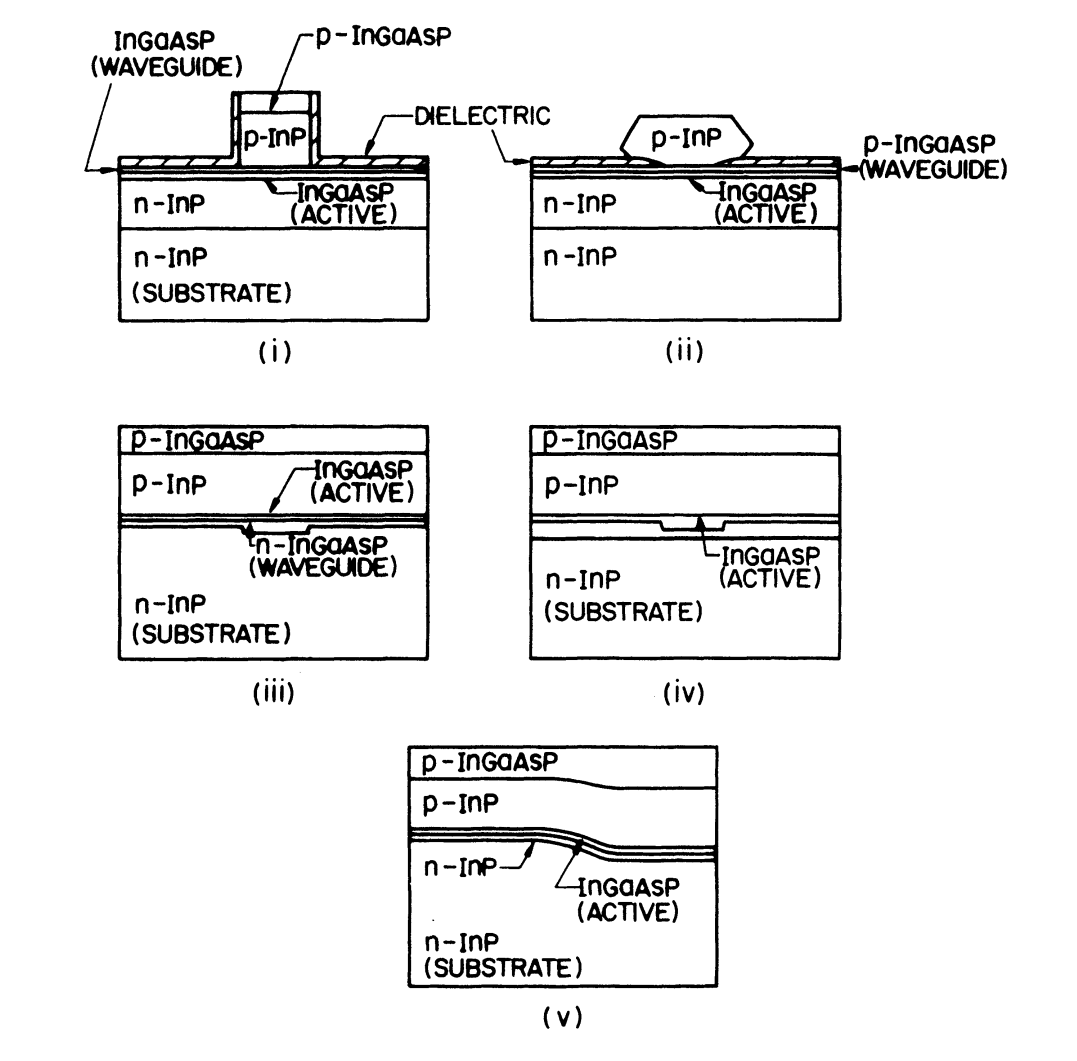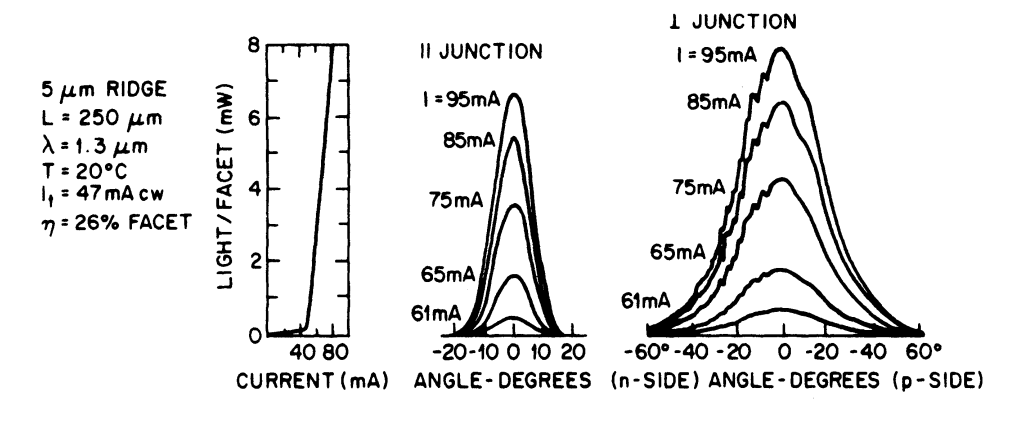# Weakly Index-Guided Lasers

This is a continuation from the previous tutorial - gain-guide lasers.

The lateral mode in gain-guided lasers is determined by the distribution of optical gain along the junction plane. The optical gain is determined by the carrier distribution that is influenced by both current spreading (due to the sheet resistance of the p cladding layer) and carrier diffusion in the active region.

As discussed in previous tutorials, gain-guided InGaAsP lasers have undesirable characteristics, such as a high threshold current and a low differential quantum efficiency, as a consequence of the carrier-induced index reduction leading to index antiguiding. The effective index depression caused by carriers at the waveguide center is $$\sim5\times10^{-3}$$.

In weakly index-guided laser structures, the laser structure is modified so as to introduce an effective index step of $$\sim10^{-2}$$, which is larger than the carrier-induced reduction.

Hence the optical mode along the junction plane in a weakly index-guided laser is essentially determined by the device structure. The transition from gain guiding to index guiding as the lateral effective index step is varied was discussed in the waveguide modes in semiconductor lasers tutorial

The design of a weakly index-guided laser requires that the thickness of at least one layer be laterally nonuniform. A number of structures, known under various names such as rib waveguide, ridge waveguide, plano-convex waveguide, channeled-substrate planar waveguide, have been used for this purpose, and some of them are shown schematically in Figure 5-14.Figure 5-14.  Schematic cross section of several types of weakly index-guided laser structures: (i) ridge-waveguide laser; (ii) heteroepitaxial ridge-overgrown laser; (iii) channeled-substrate planar waveguide laser; (iv) simple rib waveguide laser; (v) terraced-substrate laser.

For ease of reference, we group them into two categories, collectively called the ridge waveguide type and the rib waveguide type.

In the ridge waveguide type of structure, the use of a dielectric around the ridge [see schemes (i) and (ii) in Figure 5-14] inhibits current spreading in the p-InP layer.

By contrast, in the rib waveguide type of structure [see schemes (iii) and (iv) in Figure 5-14], current spreading in the p-InP layer can influence the threshold current.

Both schemes are capable, however, of providing an effective index step of $$\sim10^{-2}$$ for the lateral mode under the ridge or rib region, and the mode is essentially index-guided.

Two types of ridge waveguide lasers are shown: (i) the structure used by Kaminow et al. for InGaAsP lasers and (ii) the heteroepitaxial ridge-overgrown laser.

The difference between the two types of rib waveguide lasers shown in Figure 5-14 is that in one case the lateral index step is provided by a waveguide layer of varying thickness, while in the other case the lateral index step arises from an active layer of varying thickness. Note that the rib can also be made on the p side.

To distinguish the two cases, the structure (iv) of Figure 5-14 is sometimes referred to as the inverted rib waveguide. Similarly, structure (iii) is also called plano-convex waveguide.

Ridge Waveguide Laser

The ridge waveguide (RWG) laser has been extensively studied. Figure 5-15 shows scanning electron microscope (SEM) photomicrographs of the laser cross section.

Device fabrication involves the following steps:

1. The planar epitaxial layers are successively grown as shown in Figure 5-14 by either LPE, VPE, or MOVPE on a (100)-oriented n-InP substrate.
2. The wafer is then etched to forma ridge with a width of $$\sim5$$ μm. The 1.1-μm InGaAsP waveguide layer acts as a stop-etch layer.
3. The wafer is then processed to produce lasers using standard dielectric deposition, photolithographic, and metallization techniques.

Figure 5-15(a) shows the SEM cross section of an RWG laser showing the ridge formed by etching. Figure 5-15(b) shows the same laser with a gold pad used for p-contact.Figure 5-15(a)  SEM cross section of a ridge waveguide laser showing the ridge formed by chemical etching. The nominal width of the ridge is about 5 μm.Figure 5-15(b)  SEM cross section of the same RWG laser showing the gold pad used for p-contact.

The planar active layer of a ridge waveguide laser extends beyond the ridge, and the threshold current is affected by carrier diffusion in the active region.

Figure 5-16 shows the typical light-current characteristics of a 1.3-μm InGaAsP ridge waveguide laser. Also shown are the far-field patterns along and normal to the junction plane. Typical threshold currents of these devices are in the range of 40-60 mA.Figure 5-16.  Typical light-current (L-I) characteristics of a 1.3-μm InGaAsP ridge waveguide laser. Far fields along and normal to the junction plane are also shown.

Lateral index guiding in a ridge waveguide laser is schematically shown in Figure 5-17(a).

A fraction of the lasing mode overlaps with the dielectric, which has a considerably lower refractive index ($$\mu\approx1.83$$) than that of the InP cladding layer ($$\mu=3.22$$). This overlap introduces an effective lateral index step as discussed in detail in the waveguide modes in semiconductor lasers tutorial.

The magnitude of the lateral index step is determined by the thicknesses of the waveguide layer. For a very thick waveguide layer, the lateral index step may become so small that it is comparable to the carrier-induced index change. The performance of a ridge waveguide laser may then become very similar to that of a gain-guided laser.Figure 5-17.  Schematic representation of the optical mode in (a) ridge waveguide and (b) rib waveguide lasers. The dashed elliptical curve shows the spatial extent of the optical mode.

Rib Waveguide Laser

Rib waveguide lasers have attracted considerable attention. The threshold current of these lasers is influenced by current spreading in the p cladding layer, by carrier diffusion in the active region, and by the lateral index step. The measured threshold currents are typically in the range of 60-80 mA.

The lateral index guiding in a rib waveguide laser is schematically shown in Figure 5-17(b).

A fraction of the lasing mode overlaps with the lower-index waveguide layer on either side of the active region. This overlap introduces a lateral index step.

The effective index step depends on the width of the active region, the thickness of the waveguide region, and the thickness variation of the waveguide layer.

An excellent control of layer thicknesses is necessary for fabrication of these devices. Large values of active and waveguide layer thicknesses can reduce the lateral index step sufficiently so that the rib waveguide laser behaves as a gain-guide laser.

The next tutorial discusses in detail about strongly index-guided lasers